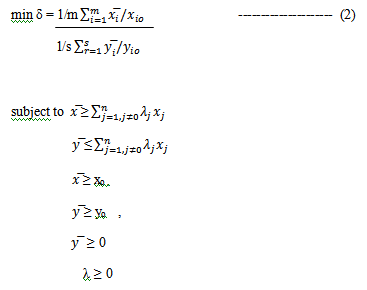# Slacks based measure or SBM analysis in DEA

Slacks based measure (SBM) is a non-radial model to solve the problem in the “additive model” developed by Charnes, Cooper, & Rhodes in 1978. This model can discriminate between efficient and inefficient Decision-Making Units (DMU). However it does not provide an efficiency measure, so a decision maker cannot interpret the performance of the DMU. Therefore “super SBM” model was introduced to determine and rank efficient DMU. The efficiency scores are dimensionless and fall between 0 and 1, thus allowing for the comparison of different DMU in terms of efficiency.

## Why SBM model?

SBM model uses “slacks” to show excess input and shortfalls in the output then it directly deals with them by maximizing these slacks. SBM analysis provides an efficiency score which is units-invariant and a monotone function of input slacks and output slacks. To rank efficiency, super SBM was introduced by Tone & Tsutsui (2001b). It is appropriate for evaluating efficiencies when inputs and outputs may change non-proportionally.

Super SBM model can be expanded by the super efficiency to the DEA models. Super efficiency rate refers to the distance between the inputs and outputs of both units. The distance is shown in variable ρ. Assuming that there exist a set of ‘n’  DMU producing the same set of outputs which consume the same set of inputs. Input and output matrix is matrix (X, Y), where (Input) X= (xij) ϵ Rmxn and (Output) Y= (yij) ϵ Rsxn.

## Equations of the SBM model

λ is a nonnegative vector in Rn. The vector S ϵ Rm and S+ ϵ Rs, shows an excess input and a short falling output, respectively.  The equation for the SBM model is as follows:Figure 1: Equation for SBM model

Suppose (ρ*, λ*,s-*, s+*) is the optimal condition for SBM analysis and (x0 , y0) is SBM efficient of DMU. When ρ* = 1, s-*=0 and s+*=0 (or there is no excess input and a short falling output). A super-efficiency model helps in ranking DMU. Below is the formula for super SBM analysis:Figure 2: Equation for super SBM model

## Challenges of super SBM model

One of the major challenges in super SBM model is that it involves solving a complex equation. This becomes more tedious when there are large units of input and outputs. It takes a lot of effort and time to estimate the efficiency of different decision-making units manually. Therefore, it is optimal to use statistical packages like DEA solver, max DEA, and others. They are more popular than manual calculations and other measures of evaluating efficiency.

## Interpretation of super SBM analysis results

This module shows the following process in determining efficiency for 10 DMU and ranks them on the basis efficiency by using Super SBM model. It uses MaxDEA software to assess efficiency. MaxDEA 7 basic is available free of cost, however, the ‘pro’ version is a paid software.

### Input and output dataset for 10 DMU

#### Exchange rates

1 68.12 3,069,559.00 2.27 30.73
2 19.99 3,488,123.37 1.70 1.04
3 29.67 3,696,265.19 5.02 0.64
4 100.63 4,138,413.19 1.35 3.67
5 45.40 4,736,995.93 3.79 0.75
6 75.63 4,984,467.88 1.72 3.15
7 53.88 6,682,944.91 3.96 1,094.85
8 24.50 13,177,694.49 3.04 6.20
9 15.92 13,544,244.95 3.54 97.60
10 13.64 23,869,948.68 2.65 1.00

By using maxDEA software and applying super SBM model, efficiency score for all DMU can be determined  and ranked. DEA-SBM model is non-radial and non-oriented and can deal with inputs and outputs individually. The purpose is to minimize the input and output slacks, resulting in this fractional program.

1 0.248082 8
2 0.541691 6
3 0.651521 5
4 1 1
5 1 1
6 0.796988 4
7 0.151898 10
8 0.359254 7
9 0.227246 9
10 1 1

## Determining the efficiency score of DMU

The results show that DMU4, DMU5, and DMU6 are the most efficient decision-making units achieving highest efficiency score of 1. The efficiency score of all other DMUs is less than 1 in DEA-SBM models. It reflects these DMUs have input excesses and output shortfalls. This analysis can be used in yearly data. The mean efficiency score for specific DMU over a period of time and year time efficiency can be evaluated too.

### Rashmi Sajwan

Research Analyst at Project Guru
Rashmi has completed her bachelors in Economic (hons.) from Delhi University and Masters in economics from Guru Gobind Singh Indrapastha University. She has good understanding of statistical softwares like STATA, SPSS and E-views. She worked as a Research Intern at CIMMYT international maize and wheat improvement centre. She has an analytical mind and can spend her whole day on data analysis. Being a poetry lover, she likes to write and read poems. In her spare time, she loves to do Dance.

#### Latest posts by Rashmi Sajwan (see all)We are looking for candidates who have completed their master's degree or Ph.D. Click here to know more about our vacancies.